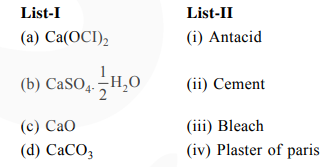# Match List-I with List-II`
Question:

Match List-I with List-IIChoose the most appropriate answer from the options given below:

1. a-i, b-iv, c-iii, d-ii

2. a-iii, b-ii, c-iv, d-i

3. a-iii, b-iv, c-ii, d-i

4. a-iii, b-ii, c-i, d-iv

Correct Option: , 3

Solution:

$\mathrm{Ca}(\mathrm{OCl})_{2}$ is Bleach.

$\mathrm{CaSO}_{4} \cdot \frac{1}{2} \mathrm{H}_{2} \mathrm{O}$ is plaster of paris.

$\mathrm{CaCO}_{3}$ is used as an antacid.

$\mathrm{CaO}$ is major component of cement.